# HSPT Math : Arithmetic

## Example Questions

### Example Question #2 : How To Solve Arithmetic Word Problems

There are 500 students at the high school. There are only two menu options: chicken or fish. If 15% of students ordered fish, how many students ordered chicken?

Possible Answers: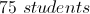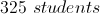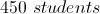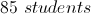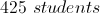Correct answer:Explanation:

If 15% of students ordered fish, then 85% of the students must have ordered chicken. Then multiply 85% or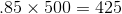.

### Example Question #22 : How To Work With Percentages

A college has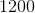students. Ifpercent of them are boys, how many girls are there?

Possible Answers: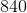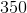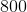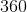Correct answer:Explanation:percent of the students are boys, sopercent of the students are girls.

Let= number of girls.

Then we can set up the following proportion: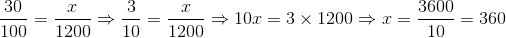### Example Question #92 : Arithmetic

In appreciation for a job well done, Loretta is being awarded a bonus equal to 7.5% of the salary she earned over the last two years. Her monthly salary over the last twelve months was $3,500 per month; she received a raise of$500 per month twelve months ago. What is the amount of the bonus?

Possible Answers: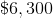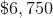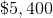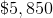Correct answer:Explanation:

Loretta earned $3,000 per month for twelve months, and$3,500 per month for the following twelve months. This is a total of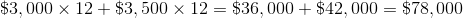earned over two years. 7.5% of this is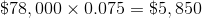,

the amount of Loretta's bonus.

### Example Question #31 : Percentages

A class has 25 students. If 60% of them are boys, how many students are girls?

Possible Answers:

10

20

8

9

15

Correct answer:

10

Explanation:

We know that 40% of the class are girls because 60% are boys. To express 40% as a fraction of the 25 total students, we set up a proportion to find the number of girls in the class.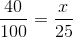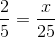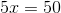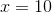When solving for, we find that there are 10 girls in the class.

### Example Question #32 : How To Work With Percentages

Fifteen percent of the students in the classroom brought their lunch from home. If 9 students brought their lunch from home, how many students are in the classroom total?

Possible Answers:

60

6

50

135

Correct answer:

60

Explanation:

You can think of the total number of students in the classroom as an unknown variable,.

You can set up an equation that includesand the information given. Normally in a percent problem, you would start with the total amount of something and multiply it by the percent to get the part of that total amount. You can set this up the same way, but the total amount is the missing information:

Total Amount x Percent = Part of Total Amount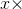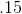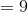You useto numerically represent 15 percent.

Now you can algebraically solve for, which will give you the total number of students in the class. You do this by dividing each side by.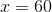Therefore there are 60 total students.

### Example Question #1 : How To Solve Arithmetic Word Problems

Candidate A getsof the votes in an election.

Candidate B gets 35% of the votes.

If 2.8 million people votes, how many more votes than Candidate A did Candidate B receive?

Possible Answers: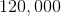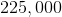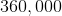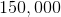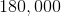Correct answer:Explanation:

First we need to getand 35% in the same terms so that we can subtract one from the other. Since 7ths are repeating decimals, it will be easiest to use fractions.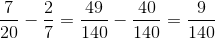.

Now we multiply this fraction by 2.8 million, which reduces nicely to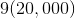, which equals 180,000.

### Example Question #3 : Coordinate Geometry

In a poll, Camille learned thatof her classmates spoke English at home,spoke Spanish, andspoke other languages. If she were to graph this data on a pie chart, what would be the degree measurement for the part representing students who speak Spanish at home?

Possible Answers: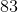Correct answer:Explanation:

In order to solve this problem, you must first solve for what percentage of the entire group comprise of Spanish-speaking students. To do this, divide the total amount of Spanish-speaking students by the total number of students.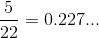Multiply this number by 100 and round up in order to get your percentage.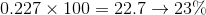Then, multiply this number times the total degrees in a circle to find out the measurement of the piece representing Spanish-speaking students on the pie chart.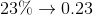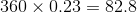Round up: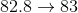### Example Question #34 : How To Work With Percentages

What isas a fraction?

Possible Answers: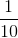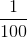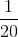Correct answer:Explanation:

The meaning of a percent is the number over 100 parts.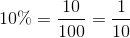### Example Question #1 : How To Find Percentage

Ifout ofstudents have contact lenses, what percentage of students have contact lenses?

Possible Answers:percentpercentpercentpercentpercent

Correct answer:percent

Explanation:

When dividingby, the reduced form is: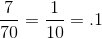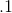is the equivalent ofpercent, which is therefore the correct answer.

### Example Question #1385 : Numbers And Operations

A class withstudents went on a field trip, but one of the students was absent.  What percentage of students in the class went on the field trip?

Possible Answers: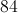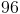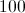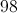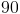Correct answer:Explanation:

The percentage of students who attended the field trip can be found by dividing the number present by the total number of students.  Here this isdivided by.  You then multiply byto get the percentage.

### All HSPT Math Resources